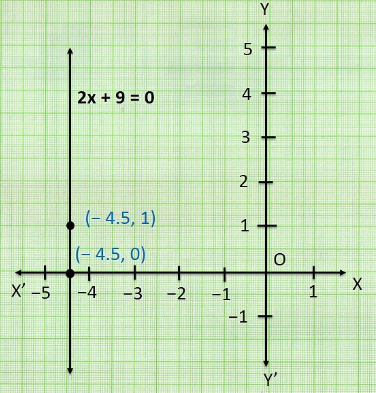# NCERT Solution Class 9 Maths Chapter 4 – Linear Equations In Two Variables

## Exercise 4.1 Page: 68

1. The cost of a notebook is twice the cost of a pen. Write a linear equation in two variables to represent this statement.

(Take the cost of a notebook to be ₹ x and that of a pen to be ₹ y)

Solution:

Let the cost of a notebook to be = ₹ x

Let the cost of a pen to be = ₹ y

According to the question,

The cost of a notebook is twice the cost of a pen.

i.e., Cost of a notebook = 2×Cost of a pen

x = 2×y

x = 2y

x-2y = 0

x-2y = 0 is the linear equation in two variables to represent the statement ‘The cost of a notebook is twice the cost of a pen’.

2. Express the following linear equations in the form ax + by + c = 0 and indicate the values of a, b and c in each case: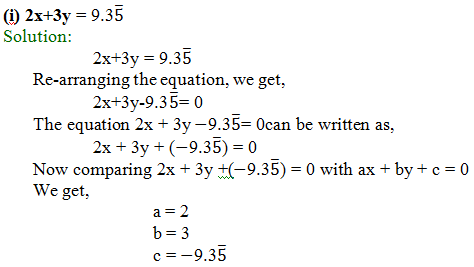(ii) x –(y/5)–10 = 0

Solution:

The equation x –(y/5)-10 = 0 can be written as,

1x+(-1/5)y +(–10) = 0

Now comparing x+(-1/5)y+(–10) = 0 with ax+by+c = 0

We get,

a = 1

b = -(1/5)

c = -10

(iii) –2x+3y = 6

Solution:

–2x+3y = 6

Re-arranging the equation, we get,

–2x+3y–6 = 0

The equation –2x+3y–6 = 0 can be written as,

(–2)x+3y+(– 6) = 0

Now comparing (–2)x+3y+(–6) = 0 with ax+by+c = 0

We get, a = –2

b = 3

c =6

(iv) x = 3y

Solution:

x = 3y

Re-arranging the equation, we get,

x-3y = 0

The equation x-3y=0 can be written as,

1x+(-3)y+(0)c = 0

Now comparing 1x+(-3)y+(0)c = 0 with ax+by+c = 0

We get, a = 1

b = -3

c =0

(v) 2x = –5y

Solution:

2x = –5y

Re-arranging the equation, we get,

2x+5y = 0

The equation 2x+5y = 0 can be written as,

2x+5y+0 = 0

Now comparing 2x+5y+0= 0 with ax+by+c = 0

We get, a = 2

b = 5

c = 0

(vi) 3x+2 = 0

Solution:

3x+2 = 0

The equation 3x+2 = 0 can be written as,

3x+0y+2 = 0

Now comparing 3x+0+2= 0 with ax+by+c = 0

We get, a = 3

b = 0

c = 2

(vii) y–2 = 0

Solution:

y–2 = 0

The equation y–2 = 0 can be written as,

0x+1y+(–2) = 0

Now comparing 0x+1y+(–2) = 0with ax+by+c = 0

We get, a = 0

b = 1

c = –2

(viii) 5 = 2x

Solution:

5 = 2x

Re-arranging the equation, we get,

2x = 5

i.e., 2x–5 = 0

The equation 2x–5 = 0 can be written as,

2x+0y–5 = 0

Now comparing 2x+0y–5 = 0 with ax+by+c = 0

We get, a = 2

b = 0

c = -5

## Exercise 4.2 Page: 70

1. Which one of the following options is true, and why?

y = 3x+5 has

1. A unique solution
2. Only two solutions
3. Infinitely many solutions

Solution:

Let us substitute different values for x in the linear equation y = 3x+5,

 x 0 1 2 …. 100 y, where y=3x+5 5 8 11 …. 305

From the table, it is clear that x can have infinite values, and for all the infinite values of x, there are infinite values of y as well.

Hence, (iii) infinitely many solutions is the only option true.

2. Write four solutions for each of the following equations:

(i) 2x+y = 7

Solution:

To find the four solutions of 2x+y =7 we substitute different values for x and y

Let x = 0

Then,

2x+y = 7

(20)+y = 7

y = 7

(0,7)

Let x = 1

Then,

2x+y = 7

(2×1)+y = 7

2+y = 7

y = 7-2

y = 5

(1,5)

Let y = 1

Then,

2x+y = 7

(2x)+1 = 7

2x = 7-1

2x = 6

x = 6/2

x = 3

(3,1)

Let x = 2

Then,

2x+y = 7

(2×2)+y = 7

4+y = 7

y =7-4

y = 3

(2,3)

The solutions are (0, 7), (1,5), (3,1), (2,3)

(ii) πx+y = 9

Solution:

To find the four solutions of πx+y = 9 we substitute different values for x and y

Let x = 0

Then,

πx+y = 9

(π0)+y = 9

y = 9

(0,9)

Let x = 1

Then,

πx +y = 9

(π×1)+y = 9

π+y = 9

y = 9-

(1, 9-)

Let y = 0

Then,

πx+y = 9

πx+0 = 9

πx = 9

x = 9/

(9/,0)

Let x = -1

Then,

πx + y = 9

(×-1) + y = 9

-+y = 9

y = 9+π

(-1,9+)

The solutions are (0,9), (1,9-), (9/,0), (-1,9+)

(iii) x = 4y

Solution:

To find the four solutions of x = 4y we substitute different values for x and y

Let x = 0

Then,

x = 4y

0 = 4y

4y= 0

y = 0/4

y = 0

(0,0)

Let x = 1

Then,

x = 4y

1 = 4y

4y = 1

y = 1/4

(1,1/4)

Let y = 4

Then,

x = 4y

x= 4×4

x = 16

(16,4)

Let y =

Then,

x = 4y

x = 4×1

x = 4

(4,1)

The solutions are (0,0), (1,1/4), (16,4), (4,1)

3. Check which of the following are solutions of the equation x–2y = 4 and which are not:

(i) (0, 2)

(ii) (2, 0)

(iii) (4, 0)

(iv) (√2, 4√2)

(v) (1, 1)

Solutions:

(i) (0, 2)

(x,y) = (0,2)

Here, x=0 and y=2

Substituting the values of x and y in the equation x–2y = 4, we get,

x–2y = 4

⟹ 0 – (2×2) = 4

But, -4 4

(0, 2) is not a solution of the equation x–2y = 4

(ii) (2, 0)

(x,y) = (2, 0)

Here, x = 2 and y = 0

Substituting the values of x and y in the equation x -2y = 4, we get,

x -2y = 4

⟹ 2-(2×0) = 4

⟹ 2 -0 = 4

But, 2 ≠ 4

(2, 0) is not a solution of the equation x-2y = 4

(iii) (4, 0)

Solution:

(x,y) = (4, 0)

Here, x= 4 and y=0

Substituting the values of x and y in the equation x -2y = 4, we get,

x–2y = 4

⟹ 4 – 2×0 = 4

⟹ 4-0 = 4

⟹ 4 = 4

(4, 0) is a solution of the equation x–2y = 4

(iv) (√2,4√2)

Solution:

(x,y) = (√2,4√2)

Here, x = √2 and y = 4√2

Substituting the values of x and y in the equation x–2y = 4, we get,

x –2y = 4

⟹ √2-(2×4√2) = 4

√2-8√2 = 4

But, -7√2 ≠ 4

(√2,4√2) is not a solution of the equation x–2y = 4

(v) (1, 1)

Solution:

(x,y) = (1, 1)

Here, x= 1 and y= 1

Substituting the values of x and y in the equation x–2y = 4, we get,

x –2y = 4

⟹ 1 -(2×1) = 4

⟹ 1-2 = 4

But, -1 ≠ 4

(1, 1) is not a solution of the equation x–2y = 4

4. Find the value of k, if x = 2, y = 1 is a solution of the equation 2x+3y = k.

Solution:

The given equation is

2x+3y = k

According to the question, x = 2 and y = 1.

Now, Substituting the values of x and y in the equation2x+3y = k,

We get,

(2×2)+(3×1) = k

⟹ 4+3 = k

⟹ 7 = k

k = 7

The value of k, if x = 2, y = 1 is a solution of the equation 2x+3y = k, is 7.

## Exercise 4.3 Page: 74

1. Draw the graph of each of the following linear equations in two variables:

(i) x+y = 4

Solution:

To draw a graph of linear equations in two variables, let us find out the points to plot.

To find out the points, we have to find the values which x and y can have, satisfying the equation.

Here,

x+y = 4

Substituting the values for x,

When x = 0,

x+y = 4

0+y = 4

y = 4

When x = 4,

x+y = 4

4+y = 4

y = 4–4

y = 0

 x y 0 4 4 0

The points to be plotted are (0, 4) and (4,0)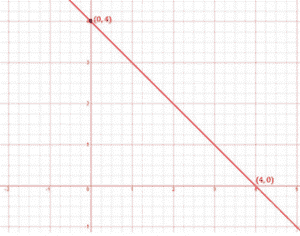(ii) x–y = 2

Solution:

To draw a graph of linear equations in two variables, let us find out the points to plot.

To find out the points, we have to find the values which x and y can have, satisfying the equation.

Here,

x–y = 2

Substituting the values for x,

When x = 0,

x–y = 2

0 – y = 2

y = – 2

When x = 2,

x–y = 2

2–y = 2

– y = 2–2

–y = 0

y = 0

 x y 0 – 2 2 0

The points to be plotted are (0, – 2) and (2, 0)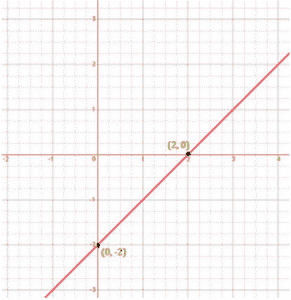(iii) y=3x

Solution:

To draw a graph of linear equations in two variables, let us find out the points to plot.

To find out the points, we have to find the values which x and y can have, satisfying the equation.

Here,

y = 3x

Substituting the values for x,

When x = 0,

y = 3x

y = 3×0

y = 0

When x = 1,

y = 3x

y = 3×1

y = 3

 x y 0 0 1 3

The points to be plotted are (0, 0) and (1, 3)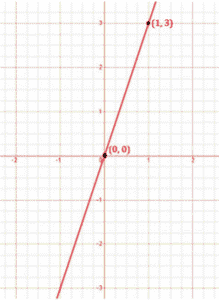(iv) 3 = 2x+y

Solution:

To draw a graph of linear equations in two variables, let us find out the points to plot.

To find out the points, we have to find the values which x and y can have, satisfying the equation.

Here,

3 = 2x+y

Substituting the values for x,

When x = 0,

3 = 2x+y

3 = 2×0+y

3 = 0+y

y = 3

When x = 1,

3= 2x+y

3 = 2×1+y

3 = 2+y

y = 3–2

y = 1

 x y 0 3 1 1

The points to be plotted are (0, 3) and (1, 1)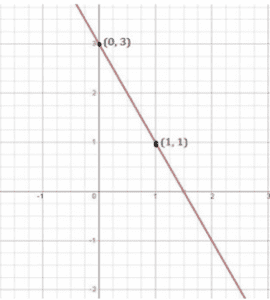2. Give the equations of two lines passing through (2, 14). How many more such lines are there, and why?

Solution:

We know that infinite number of lines passes through a point.

Equation of 2 lines passing through (2,14) should be in such a way that it satisfies the point.

Let the equation be, 7x = y

7x–y = 0

When x = 2 and y = 14

(7×2)-14 = 0

14–14 = 0

0 = 0

L.H.S = R.H.S

Let another equation be, 4x = y-6

4x-y+6 = 0

When x = 2 and y = 14

(4×2–14+6 = 0

8–14+6 = 0

0 = 0

L.H.S = R.H.S

Since both the equations satisfies the point (2,14), than say that the equations of two lines passing through (2, 14) are 7x = y and 4x = y-6

We know that, infinite number of line passes through one specific point. Since there is only one point (2,14) here, there can be infinite lines that passes through the point.

3. If the point (3, 4) lies on the graph of the equation 3y = ax+7, find the value of a.

Solution:

The given equation is

3y = ax+7

According to the question, x = 3 and y = 4

Now, Substituting the values of x and y in the equation 3y = ax+7,

We get,

(3×4) = (a×3)+7

⟹ 12 = 3a+7

⟹ 3a = 12–7

⟹ 3a = 5

⟹ a = 5/3

The value of a, if the point (3,4) lies on the graph of the equation 3y = ax+7 is 5/3.

4. The taxi fare in a city is as follows: For the first kilometer, the fare is 8 and for the subsequent distance it is 5 per km. Taking the distance covered as x km and total fare as  y, write a linear equation for this information, and draw its graph.

Solution:

Given,

Total distance covered = x

Total fare = y

Fare for the first kilometer = 8 per km

Fare after the first 1km = 5 per km

If x is the total distance, then the distance after one km = (x-1)km

i.e., Fare after the first km = 5(x-1)

According to the question,

The total fare = Fare of first km+ fare after the first km

y = 8+5(x-1)

y = 8+5(x-1)

y = 8+5x – 5

y = 5x+3

Solving the equation,

When x = 0,

y = 5x+3

y = 5×0+3

y = 3

When y = 0,

y = 5x+3

o = 5x+3

5x = -3

x = -3/5

 x y 0 3 -3/5 0

The points to be plotted are (0, 3) and (-3/5, 0)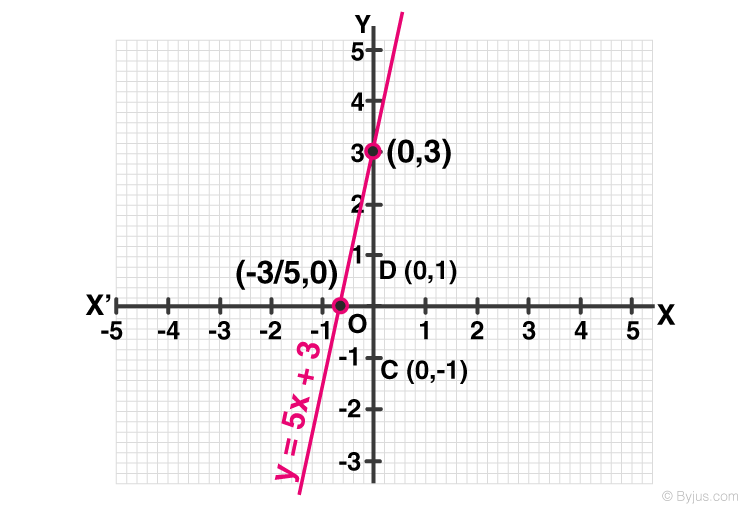5. From the choices given below, choose the equation whose graphs are given in Fig. 4.6 and Fig. 4.7.

For Fig. 4. 6

(i) y = x

(ii) x+y = 0

(iii) y = 2x

(iv) 2+3y = 7xSolution:

The points given in the figure 4.6 are (0,0), (-1,1), (1,-1)

Substituting the values for x and y from these points in the equations, we get,

(i) y = x

(0,0) ⟹ 0 = 0

(-1, 1) ⟹ -1 ≠ 1 ————————— equation not satisfied

(1, -1) ⟹ 1≠ -1 ————————— equation not satisfied

(ii) x+y = 0

(0,0) ⟹ 0+0 = 0

(-1, 1) ⟹ -1+1 = 0

(1, -1) ⟹ 1+(-1) =0

(iii) y = 2x

(0,0) ⟹ 0 = 2×0

0 = 0

(-1, 1) ⟹ 1 = 2×(-1)

1≠ -2 ————————— equation not satisfied

(1, -1) ⟹ -1 = 2×1

-1 ≠ 2 ————————— equation not satisfied

(iv) 2+3y = 7x

(0,0) ⟹ 2+(30) = 7×0

2 ≠ 0 ————————— equation not satisfied

(-1, 1) ⟹ 2+(3×1) = 7×-1

5 ≠ -7 ————————— equation not satisfied

(1, -1) ⟹ 2+(3×-1) = 7×1

-1 ≠ 7 ————————— equation not satisfied

Since, only equation x+y = 0 satisfies all the points, the equation whose graphs are given in Fig. 4.6 is

x+y = 0

For Fig. 4. 7

(i) y = x+2

(ii) y = x–2

(iii) y = –x+2

(iv) x+2y = 6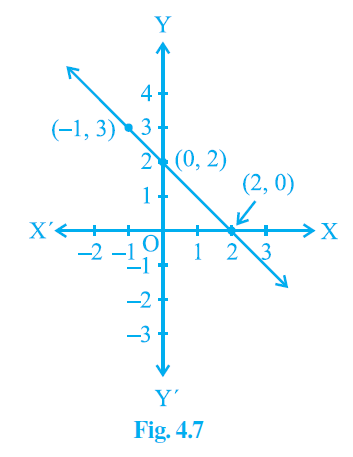Solution:

The points given in the figure 4.7 are (0,2), (2,0), (-1,3)

Substituting the values for x and y from these points in the equations, we get,

(i) y = x+2

(0,2) ⟹2 = 0+2

2 = 2

(2, 0) ⟹ 0= 2+2

0 ≠ 4 ————————— equation not satisfied

(-1, 3) ⟹ 3 = -1+2

3 ≠ 1 ————————— equation not satisfied

(ii) y = x–2

(0,2) ⟹ 2 = 0–2

2 ≠ -2 ————————— equation not satisfied

(2, 0) ⟹ 0 = 2–2

0= 0

(-1, 3) ⟹ 3= –1–2

3 ≠ –3 ————————— equation not satisfied

(iii) y = –x+2

(0,2) ⟹ 2 = -0+2

2 = 2

(2, 0) ⟹ 0 = -2+2

0 = 0

(-1, 3) ⟹ 3= -(-1)+2

3 = 3

(iv) x+2y = 6

(0,2) ⟹ 0+(2×2) = 6

4 ≠ 6 ————————— equation not satisfied

(2, 0) ⟹ 2+(2×0) = 6

2 ≠ 6 ————————— equation not satisfied

(-1, 3) ⟹ -1+(2×3) = 6

5 ≠ 6 ————————— equation not satisfied

Since, only equation y = –x+2satisfies all the points, the equation whose graphs are given in Fig. 4.7 is

y = –x+2

6. If the work done by a body on application of a constant force is directly proportional to the distance travelled by the body, express this in the form of an equation in two variables and draw the graph of the same by taking the constant force as 5 units. Also read from the graph the work done when the distance travelled by the body is

(i) 2 units

(ii) 0 unit

Solution:

Let the distance traveled by the body be x and the force applied on the body be y.

It is given that,

The work done by a body is directly proportional to the distance travelled by the body.

According to the question,

y ∝ x

y = 5x (5 is a constant of proportionality)

Solving the equation,

(i) when x = 2 units,

then y = 5×2 = 10 units

(2, 10)

(ii) when x = 0 units,

then y = 5×0 = 0 units.

(0, 0)

The points to be plotted are (2, 10) and (0, 0)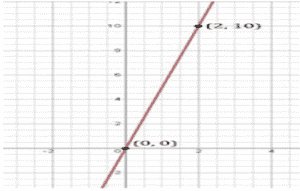7. Yamini and Fatima, two students of Class IX of a school, together contributed ₹ 100 towards the Prime Minister’s Relief Fund to help the earthquake victims. Write a linear equation which satisfies this data. (You may take their contributions as ₹ x and ₹ y.) Draw the graph of the same.

Solution:

Let Yamini’s donation be ₹x and Fatima’s donation be ₹y

According to the question;

x+y = 100

We know that,

when x  = 0 , y = 100

when x = 50, y = 50

when x = 100, y = 0

The points to be plotted are (0,100), (50,50), (100,0)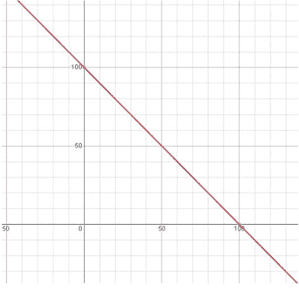8. In countries like USA and Canada, temperature is measured in Fahrenheit, whereas in countries like India, it is measured in Celsius. Here is a linear equation that converts Fahrenheit to Celsius: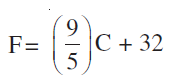(i) Draw the graph of the linear equation above using Celsius for x-axis and Fahrenheit for y-axis.

(ii) If the temperature is 30°C, what is the temperature in Fahrenheit?

(iii) If the temperature is 95°F, what is the temperature in Celsius?

(iv) If the temperature is 0°C, what is the temperature in Fahrenheit and if the temperature is 0°F, what is the temperature in Celsius?

(v) Is there a temperature which is numerically the same in both Fahrenheit and Celsius? If yes, find it.

Solution:

(i) According to the question,

F = (9/5)C + 32

Solving the equation,

We get,

When C = 0, F = 32

When C = -10 , F = 14

The points to be plotted are (0, 32), (-10, 14)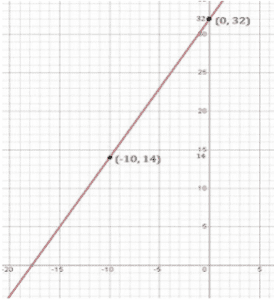(ii) When C = 30,

F = (9/5)C +32

F = (9×30)/5+32

= (9×6)+32

= 54+32

= 86oF

(iii) When F = 95,

95 = (9/5)C +32

(9/5)C = 95-32

(9/5)C =63

C = (63×5)/9

=35oC

(iv) When C = 0,

F = (9/5)C +32

F = (9×0)/5 +32

=0+32

=32oF

When F = 0,

0 = (9/5)C+32

(9/5)C = 0-32

(9/5)C = -32

C = (-32×5)/9

=-17.7777

=-17.8oC

(v) When F = C,

C = (9/5)C+32

C – (9/5)C = 32

(5-9)C/5 =32

(-4/5)C = 32

(-4/5)C = (-32×5)/4

= – 40oC

Hence, -40o is the temperature which is numerically the same in both Fahrenheit and Celsius.

## Exercise 4.4 Page: 77

1. Give the geometric representations of y = 3 as an equation

(i) in one variable

(ii) in two variables

Solution:

1. In one variable, y = 3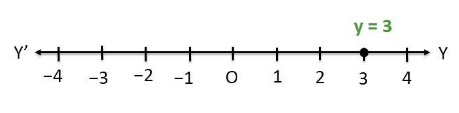(ii) In two variable, 0x+y = 3

When x = 0, y = 3

When x = 1, y = 3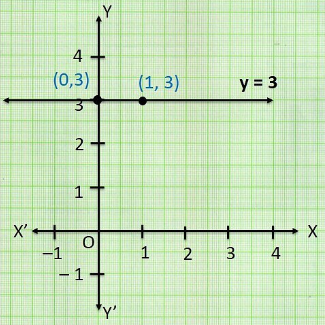2. Give the geometric representations of 2x+9 = 0 as an equation

(i) in one variable

(ii) in two variables

Solution:

(i) In one variable,

2x+9 = 0

2x = -9

x = -9/2

x = -4.5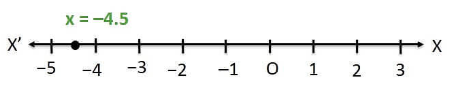(ii) In two variables,

2x+9 = 0

2x+0y+9 = 0

When y = 0, x = -4.5

When y = 1, x = -4.5